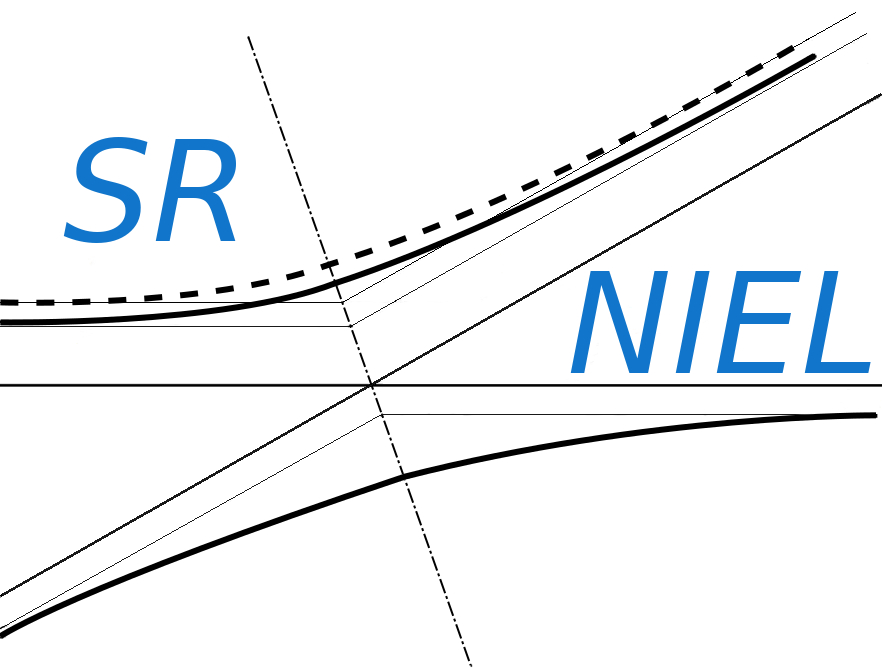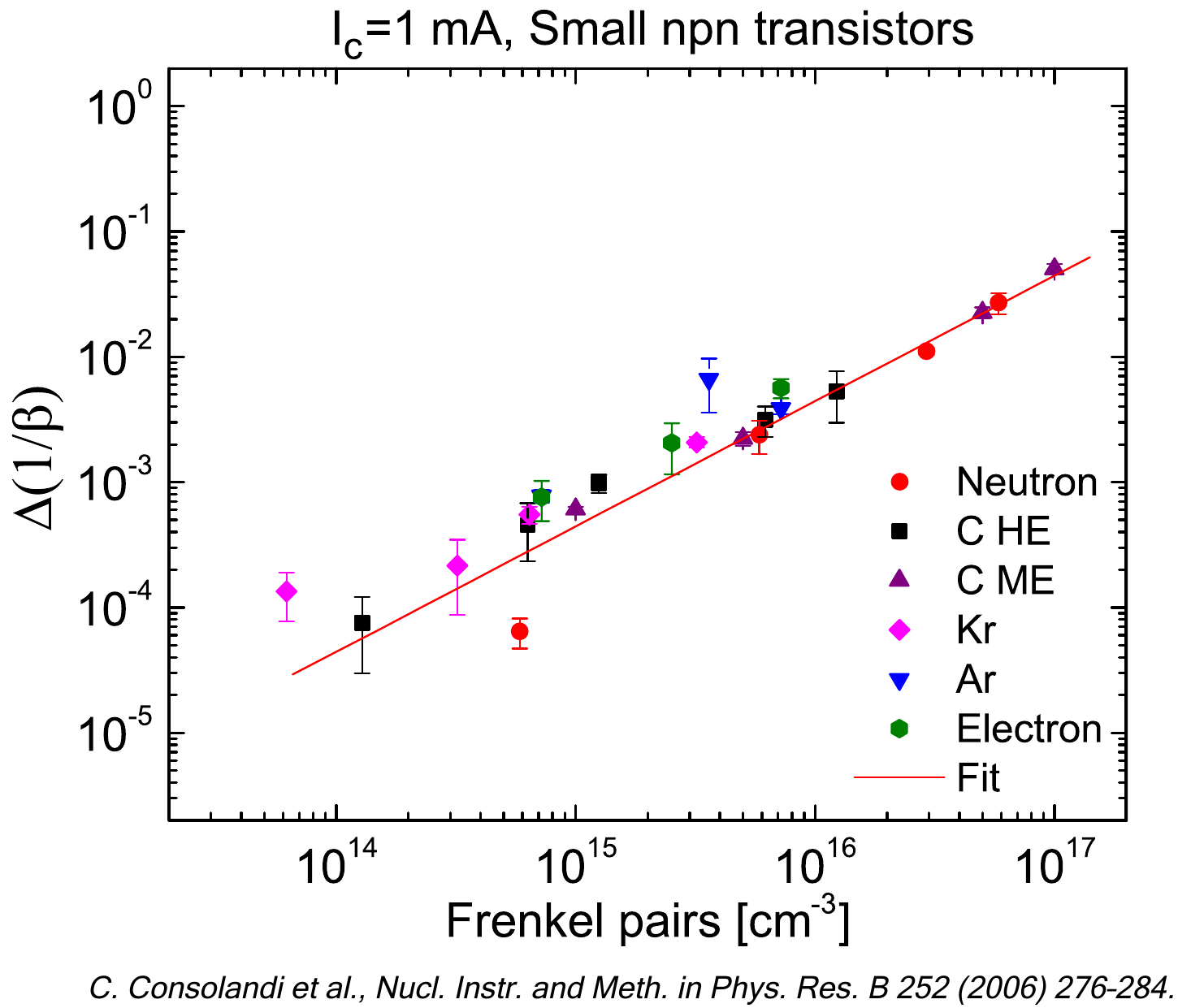SR-NIEL – 7 Screened Relativistic (SR) Treatment for NIEL Dose Nuclear and Electronic Stopping Power Calculator (version 8.0)This is the Total NIEL Dose Web application for neutrons.

### How to use this Total NIEL Dose Calculator for Neutrons

This tool calculates the total dose imparted, given the spectral fluence of neutrons as a function of the energy and the total fluence. Doses are evaluated using ASTM-722 (2009, 2014 and 2019) damage functions for neutrons in Si and GaAs.

The input parameters and options for the tool are described below.
When the input form has been completed, pressing the "CALCULATE" button will start the calculation and open the "Results" page (allow for pop-up in your browser settings).
The result page will be also linked at the bottom of the calculator page.

### Input Parameters:

- Damage function
- Target material (Si or GaAs)
- Spectral Fluence vs Energy
- Interpolating Method
- Spectrum Parameters.

### Damage Function

Here it is possible to select the damage function corresponding to different ASTM-722 (2009, 2014 and 2019) standards.

### Target Material

In this section it is possible to specify the target material selecting Si or GaAs. The displacement threshold energy will change accordingly (25 eV for Si and 10 eV for GaAs).

### Spectral Fluence

This section define the points of the spectral fluence as a function of energy.
The input format is one point per line (Energy - Flux , separated by a space or tab); it is also possible to copy and paste values.
The spectral fluence is normalized to the requested fluence value or, as default, to the total fluence obtained from provided spectrum.

### Interpolating Method

This section define the interpolating method used to obtain the Spectral fluence at the ASTM E722 (2009 & 2014) energy values.
It is possible to select Step, Linear o Spline interpolating function.

### Spectrum Parameters

This section define the spectrum parameters that are used to obtain the Spectral Dose at the ASTM E722 (2009 & 2014) energy values.
They are:
- Requested Fluencetotal: Total fluence to which the integral of the neutron spectral fluence is normalized.
- Elow : Lowest energy above which the total fluence is evaluated.
- Emin : Lowest energy from which the NIEL dose calculation is performed.

The spectral Dose is integrated to obtain the total Dose. The integral is performed from Emin and from Elow and the two values are displayed in the result table.
The spectral dose is normalized to the requested fluence value or, as default, to that corresponding to the total fluence obtained from the provided spectrum.

### Result

The result page contains the input parameters and the results graphs and table.
The graph of the spectral fluence, which contain the input points and the interpolated values, is given.
Also, the graph of the spectral dose as a function of energy is given.
The result table contains the Dose (in [Mev g-1] and [Gy]) calculated with E>Emin and with E>Elow.